Engineering Physics

Lab Report #4

## PH - 261 - 11

Projectile Motion 2

Andy Buettner

Mr. Huttlin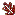Objective

This lab is to study the projectile motion in which it starts at height = 0 and ends at the same height. The projectile, however, is launched at one of three fixed angles that are not = 0o.Materials

1. Spring launcher with built in protractor (same as lab 3)

2. Ball (same as lab 3)

3. Paper

4. Measuring apparatus

1. Meter stick

2. Tape Measure

5. Ink paper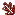Procedure

1. Set launcher from lab #3 on the floor.

2. Set the launcher to an angle of 30o.

3. Launch the ball and estimate the area of impact and the maximum height.

4. Place paper covered by ink paper over the area where the ball will hit.

5. Place a meter stick approximately where the height of the ball will be the maximum.

6. Launch the ball and use the meter stick to estimate the height obtained by the ball.

7. Record the mark made by the ball on the paper and the height obtained by the ball.

8. Repeat steps 6 and 7 two additional times.

9. Set the launcher to an angle of 45o.

10. Repeat Steps 3 through 8.

11. Set the launcher to and angle or 60o.

12. Repeat Steps 3 through 8 again.

13. Measure the distance between the launcher and the paper.

14. Measure the individual distances between the edge of the paper and the marks to find the total distance.Data

1

2

3

Maximum Height

69cm

66cm

67cm

358.8cm

354.3cm

348.2cm

1

2

3

Maximum Height

115cm

112cm

111cm

376.2cm

370.2cm

368.1cm

# III) Table 3: Trials at 60o

### Trial #

1

2

3

Maximum Height

156cm

172cm

159cm

#### Total Distance Traveled

313.6cm

335.7cm

317.5cm

IV) Diagram 1: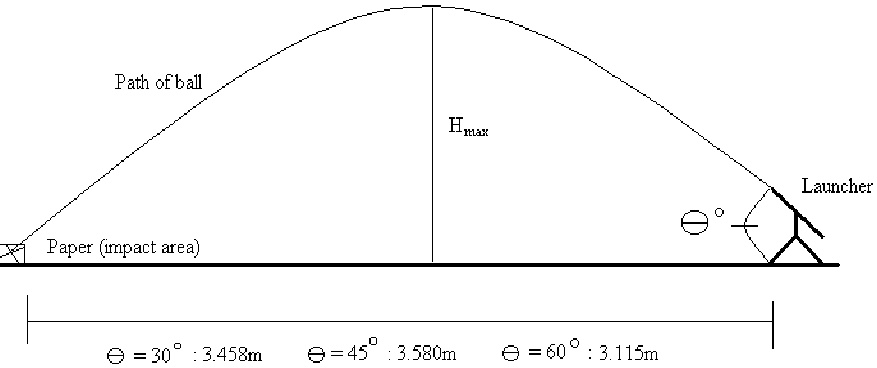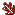Analysis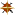Notice: All calculations done on attached sheets!Result Table 30 o 45 o 60o Ravg 353.8cm 371.5cm 322.3cm Vm 6.33m / s 6.03m / s 6.04m / s Havg (Observed) 67.3cm 112.7cm 162.3cm Havg (Calculated) 51.1cm 92.9cm 139.5cm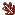Conclusions

First, I would like to mention that this lab is not as accurate as lab 3 because there were no calculations for the initial height of the ball or the decreased muzzle velocity due to the acceleration of gravity. From this lab one could conclude that a projectile will follow a parabolic path through the air and will obtain maximum distance when launched at an angle of 45o, but maximum height.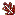Answer to Lab Questions

1) Q: What are the basic assumptions that are made about the vertical and horizontal components in order to solve a projectile motion problem?

A: First, in this experiment we assume that the initial height and distance is 0. Also, we assume that air resistance is negligible.

2) Q: Explain how air resistance affects the height and range of a projectile.

A: Air resistance decreases both values as well as causes the projectile to go off path.

3) Q: Compare the initial velocity with the ones in Lab 3. Why does the difference occur?

A: Two reasons: First of all, there was no calculation to include that the initial height was 0. Second, gravity was working against the accelerator.

4) Q: What angle gives the maximum range?

A: An angle of 45o.

• Justified on paper.